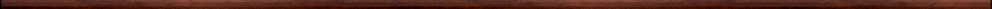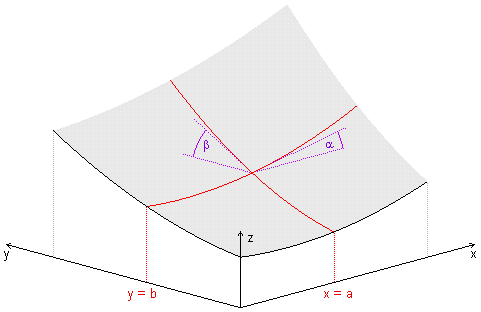Maple worksheets on multi-variable calculusMore calculus topics:

They are all compatible with Classic Worksheet Maple 10.Functions of several variables - sevvar.mws

• Examples of functions of two variables
• Functions of three variables

Partial derivatives for functions of two variables - pardiff.mws

• Definition of partial derivatives
• Examples of partial derivatives
• The function f(x,y) = x y
• Geometrical interpretation of partial derivatives
• 2nd order partial derivatives

The chain rule for functions of two variables - chainrule.mws

• The chain rule
• Chain rule examples
• Geometrical illustration of the chain rule
• Implicit differentiation

The tangent plane to a surface and differentiability of a function of two variables tanplane.mws

• Linear approximation of a function of two variables and tangent planes
• Constructing a tangent plane via tangent lines to two cross-section curves
• Using a tangent plane to help in illustrating partial derivatives
• The concept of differentiability for functions of two variables
• A general formula for the tangent plane to the graph of a function of two variables

Cubic surfaces and cubic approximations for functions of two variables - cubicsurf.mws

• Cubic surfaces
• Cubic approximation examples

• Quadratic forms - determining the nature of the critical points at the origin
• An alternative way of deriving the classification criterion for the nature of the critical point at the origin

Critical points on the graph of a function of two variables - critpts.mws

• Critical points: local maxima and minima and saddle points
• Classifying the critical points of a function of two variables

Classifying the critical points on the graph of a function of two variables-an example - critptex.mws

Classifying the critical points on the graph of a function of two variables-an example - critptex2.mws

Classifying the critical points on the graph of a function of two variables-an example - critptex3.mws

Classifying the critical points on the graph of a function of two variables-an example - critptex4.mws

Limits of functions of two variables - limits.mws

• Limits and continuity of functions of two variables

Mixed second order partial derivatives of a function of two variables - mixedPD.mws

• An example of a function of two variables for which the mixed second order partial derivatives are not equal

Partial derivatives of functions of three variables - pardiff2.mws

• Partial derivatives of functions of three variables
• The chain rule for functions of three variables
• Chain rule examples

The gradient of functions of 2 and 3 variables - directional derivatives - dirderiv.mws

• The gradient of a function of two variables
• The gradient of a function of three variables
• Graphical examples
• The directional derivative of a function of three variables
• The direction of the gradient vector at a point

A directional derivative example - dirderivex.mws

• A directional derivative example

Tangent planes to level surfaces of functions of three variables - tanplane2.mws

• The chain rule in terms of gradient
• The gradient vector as a normal vector to level surfaces
• ExamplesTop of page

Main index